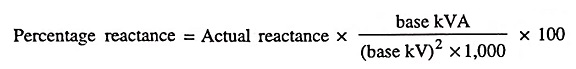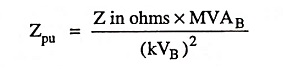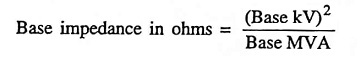## Representation of Power System Components Interview Questions and Answers:

1. What is meant by percentage reactance ?

Ans. Percentage reactance can be defined as the voltage drop across the given reactance expressed as percentage of normal voltage when carrying full-load current pertaining to normal rating.

2. Which of the electrical quantities are chosen as base values ?

Ans. For common representation, base kVA (or MVA) and base voltage (kV) are chosen as base values.

3. What is meant by per unit value of any quantity ?

Ans. Per unit value of any quantity is defined as the ratio of the actual value of the quantity in any unit to the base or reference value in the same unit.

4. What is the relationship between base kVA, base kV and percentage reactance ?

Ans.5. Write expression for per unit impedance (Zpu) in a 3-phase system.

Ans.6. Why the per unit method is considered superior to per cent method for short-circuit calculations ?

Ans. Per cent value is not convenient for use as the factor of 100 has to be carried in computations.

7. Define base impedance of a power system element and write an expression for it in terms of base MVA and kV.

Ans. Base impedance is defined as the ratio of base voltage in volts and base current in amperes and it is expressed in terms of base MVA and kV as follows :8. What is meant by base quantities ?

Ans. For common representation, the quantities (voltage, current, power or impedance) chosen as common or reference quantities are called the base quantities.

9. Define per unit power.

Ans. Per unit power is defined as the ratio of actual value of power in any unit (in kVA, MVA, kW or MW) to the base or reference value of power in the same unit.

10. Define pu impedance.

Ans. Per unit impedance is defined as the ratio of voltage drop across the given impedance to the normal voltage when carrying full-load current pertaining to normal rating.

11. What is relation between percentage impedance and pu impedance ?

Ans. Percentage impedance = PU impedance x 100

Scroll to Top